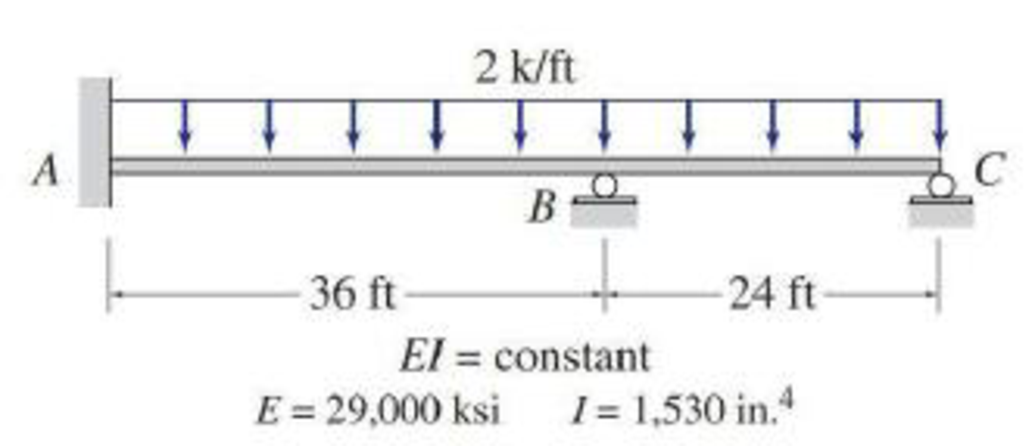# Solve Problem 15.4 for the loading shown in Fig. P15.4 and the support settlements of 1 in. at B and 1 4 in. at C . FIG. P15.4, P15.7

#### Solutions

Chapter
Section
Chapter 15, Problem 7P
Textbook Problem
54 views

## Solve Problem 15.4 for the loading shown in Fig. P15.4 and the support settlements of 1 in. at B and 1 4 in. at C.FIG. P15.4, P15.7

To determine

Find the reaction and plot the shear and bending moment diagram.

### Explanation of Solution

Fixed end moment:

Formula to calculate the fixed moment for UDL is WL212.

Calculation:

Consider the flexural rigidity EI of the beam is constant.

Show the free body diagram of the entire beam as in Figure 1.

Refer Figure 1,

Calculate the fixed end moment for AB.

FEMAB=2×36212=216kft

Calculate the fixed end moment for BA.

FEMBA=2×36212=216kft

Calculate the fixed end moment for BC.

FEMBC=2×24212=96kft

Calculate the fixed end moment for CB.

FEMCB=2×24212=96kft

Chord rotations:

Show the free body diagram of the chord rotation due to support reaction of the beam as in Figure 2.

Calculate the chord rotation of the beam AB.

ψAB=1in.36ft×12in.ft=0.002315

Calculate the chord rotation of the beam BC.

ψBC=(10.25)in.24ft×12in.ft=0.002604

Calculate the slope deflection equation for the member AB.

MAB=2EIL(2θA+θB3ψAB)+FEMAB

Here, θA is the slope at the point A and θB is the slope at the point B.

Substitute 29,000ksi for E, 1530in.4 for I, 0.002315 for ψAB, 0 for θA, 36ft for L and 216kft for FEMAB.

MAB=2×29,000×1,53036×144(2(0)+θB3(0.002315))+216=17,118.0556θB+118.89+216=17,118.0556θB+334.9 (1)

Calculate the slope deflection equation for the member BA.

MBA=2EIL(2θB+θA3ψBA)+FEMBA

Substitute 29,000ksi for E, 1530in.4 for I, 0.002315 for ψAB, 0 for θA, 36ft for L and 216kft for FEMBA.

MBA=2×29,000×1,53036×144(2θB+03(0.002315))216=34,236.11θB+118.89216=34,236.11θB97.11 (2)

Calculate the slope deflection equation for the member BC.

MBC=3EIL(θB+θCψBC)+FEMBC+FEMBC2

Substitute 29,000ksi for E, 1530in.4 for I, 0.002604 for ψBC, 0 for θC, 24ft for L and 96kft for FEMBC.

MBC=3×29,000×1,53024×144(θB+0(0.002604))+96+962=38,515.625θB100.3+144=38,515.625θB+43.7 (3)

Calculate the slope deflection equation for the member CB.

MCB=0

Write the equilibrium equation as below.

MBA+MBC=0

Substitute equation (1) and equation (2) in above equation.

34,236.11θB97.11+38,515.625θB+43.7=072,751.732θB53.41=0θB=53.4172,751.732θB=0.000734kft2

Substitute 0.000734kft2 for θB in equation (1).

MAB=17,118.0556(0.000734)+334.9=347.5kft

Substitute 0.000734kft2 for θB in equation (2).

MBA=34,236

### Still sussing out bartleby?

Check out a sample textbook solution.

See a sample solution

#### The Solution to Your Study Problems

Bartleby provides explanations to thousands of textbook problems written by our experts, many with advanced degrees!

Get Started

Find more solutions based on key concepts
For the stopping sight distance problem of Figure 18.12, estimate the stopping distance for speed of 27 mph, us...

Engineering Fundamentals: An Introduction to Engineering (MindTap Course List)

List methods for securing against hardware theft and vandalism.

Enhanced Discovering Computers 2017 (Shelly Cashman Series) (MindTap Course List)

Band saw cutting speeds are given in _________.

Precision Machining Technology (MindTap Course List)

What is schema, and how is it used?

Fundamentals of Information Systems

What is stream processing, and why is it sometimes necessary?

Database Systems: Design, Implementation, & Management

Describe the Big Data phenomenon.

Database Systems: Design, Implementation, & Management

If your motherboard supports ECC DDR3 memory, can you substitute non-ECC DDR3 memory?

A+ Guide to Hardware (Standalone Book) (MindTap Course List)

Why is it important to not weld when everything is wet?

Welding: Principles and Applications (MindTap Course List)